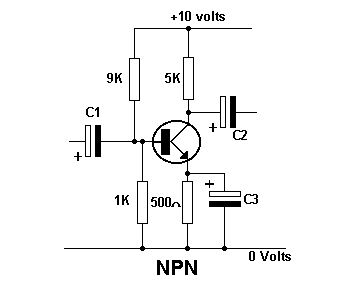Home > Electronic Tutorials > Junction Transistors Tutorial > Typical Circuit Values Tutorial

Junction Transistors Electronic Tutorials

# Typical Circuit Values TutorialThe base voltage Vb, is set by potential divider 9K and 1K = 1 volt (ignoring Ib).

The emitter voltage Ve,  is Vb - 0.6 = 0.4 volts.

The emitter current is Ve / Re = 0.0008A.

The voltage across the 5K load resistor is 5000 x .0008 = 4 volts.

Therefore the collector voltage is 10 volts - 4 volts = 6 volts.

The power dissipated by the transistor is the voltage across the transistor multiplied by the current through it. = 5.6 volts x 0.0008A = 0.00448 watts.